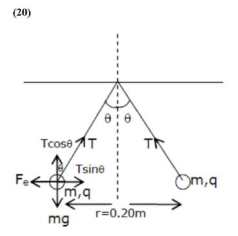# Two small spheres each of mass 10 mg

Question:

Two small spheres each of mass $10 \mathrm{mg}$ are suspended from a point by threads $0.5 \mathrm{~m}$ long. They are equally charged and repel each other to a distance of $0.20 \mathrm{~m}$. Then charge on each of the sphere is $\frac{a}{21} \times 10^{-8} \mathrm{C}$. The value of ' $\mathrm{a}$ ' will be

Solution:$T \sin \theta=\frac{k q^{2}}{r^{2}}$

$T \cos \theta=m g$

$\tan \theta=\frac{\mathrm{kq}^{2}}{\mathrm{mgr}^{2}}$

$q^{2}=\frac{\tan \theta m g r^{2}}{k}$

$\because \tan \theta=\frac{0.1}{0.5}=\frac{1}{5}$

$q^{2}=\frac{1}{5} \times \frac{10 \times 10^{-6} \times 10 \times 0.2 \times 0.2}{9 \times 10^{9}}$

$q=\frac{2 \sqrt{2}}{3} \times 10^{-8}$

after comparison from the given equation $a=20$